Being Bayesian and thinking deep: time-series prediction with uncertaintyPicture taken from here

In this post we are going to tackle the problem of time series prediction and more specifically, financial time series prediction. Formally, we wish to build models to anticipate the next time step (t+1) or next k time steps (t+k) given a time series with t time steps of data. This could be done for both uni-variate (single variable) or multi-variate (multiple variable) data. Let’s first keep things simple and look at a uni-variate prediction problem, i.e., we won’t consider the advanced case of modeling dependencies between multiple different time series.

Time series prediction has a long history. Some of the popular classical methods in the field are:

• Auto-regression
• Moving-average
• Exponential-smoothing
• Trend following.

Many variations based on the above methods have been developed over the years. In order to keep the post succinct we will not discuss all these classical methods in depth, however the inquisitive reader may refer to this book. Most classical methods create linear functions on transformations of the actual time series in order to make future time step prediction. Unlike these methods, recent models have applied deep neural networks with inherently less restrictions on the input, while being able to explore underlying nonlinear relationships in the data. Some time series, like in the case of financial data, are inherently non-stationary, i.e. the statistics of the data changes over time. This makes it difficult to model and challenging to naively apply neural network function approximation (a neural network typically learns a function that maps inputs or observations to target or predictions) which may not be suitable to cope with such non-stationarity and mismatch in data distributions.

Alternative to neural network based models, stochastic process based models like Gaussian process (GP) may also be a good choice. They make use of prior knowledge and learn a distribution over functions rather than a single function approximation. This makes GP models Bayesian as they involve constructing a prior distribution (here over functions directly rather than over parameters) and updating this distribution by conditioning on the actual data. This is particularly useful for financial data because of its volatile nature. The price of a financial asset is sensitive to many external or internal events like policy reforms, intrinsic turbulence in the market, news sentiments and natural disasters. Now with a distribution of functions, the risk or uncertainty can be embedded in the standard deviation of the model’s predictions. This makes us better informed while making decisions and forming strategies based on these predictions.

Due to the highly non-stationary nature of financial time series, the model we create, ideally must also evolve with time as the relationship between the past and future is unlikely to remain stationary. In this post, we will discuss and evaluate the application of GPs and their deep variant called Deep Gaussian processes (DGPs) for financial time series prediction with uncertainty.

How do we formulate a Gaussian Process?

Let’s reformulate the problem so that the GP paradigm can be applied to it, given the past data points (xₜ, yₜ) with t ∈ {1, …, n}, we first understand the underlying relationship between x and y, then we can obtain the prediction y* given some new x*. As mentioned earlier, we only look at uni-variate time series, here x is composed of the past k time steps observations of a single time-series and k is a hyper-parameter. GPs find a distribution over the possible functions that are consistent with the observed data.

Definition ( Bishop’s Pattern Recognition and Machine Learning): Let f be a function mapping some input space 𝕏 to ℝ and f be an n-dimensional vector of function values evaluated at n points xₜ∈𝕏, then p(f) is a Gaussian process if for any finite subset {x₁, …, xₙ}⊂ 𝕏, the marginal distribution over that finite subset p(f) has a multivariate Gaussian distribution. A GP is fully specified by its mean and co-variance.

The typical idea for a GP to make prediction is to first set a Gaussian prior with the distribution function f, with a given set of data points (xₜ, yₜ) which is assumed to contain Gaussian noise, the posterior is then obtained by marginalizing the product of likelihood p(f*|f) and prior p(f) over f. Despite this simple derivation, GP requires O(n³) computations to obtain the prediction mean and standard deviation which is expensive with large observation set. A few sparse versions of GP, such as Sparse Gaussian process regression (SGPR) and Sparse variational Gaussian process (SVGP) have been introduced and the main idea is to compute the approximation of p(f*, f) by assuming the independence of f* and f given some inducing variables and computing the predictive probability through variational inference, the computational cost can be reduced to O(nm²) where m is the number of inducing points.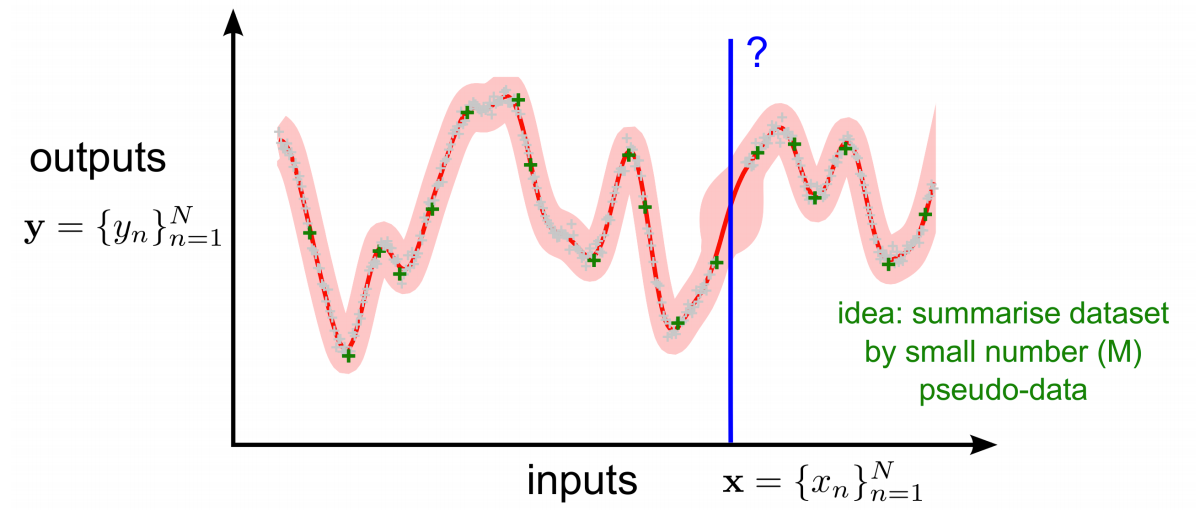Figure 1: SGPR (image from here)

The single layer GP models mentioned above are limited by the expressiveness of the kernel function in the selected prior. This limitation could be solved by introducing a hierarchical composition of GPs, known as Deep Gaussian Process (DGP). A DGP is a deep network in which each layer is modeled by a GP. This provides the possibility to model highly nonlinear functions for complex data sets. Due to the hierarchical structure, it often requires very few hyper-parameters in each layer GP. Many DGP models assume independence between layers to perform inference which is not realistic. A recently proposed method Doubly stochastic variational inference for deep Gaussian process (DDGP) uses sparse variational inference to simplify the correlations within layers and maintain the correlations between layers.

Applying GP and DGP models for online time-series prediction

Online setting: Now we look at the performance of Sparse GP, DDGP models on time series prediction within a continuous learning setting. In order to formulate these models within an online framework, we update parameters of the model continuously over a moving window. This will make predictions more timely and robust. The window is shifted forward in a walk-forward step equal to the number of data points in the testing set. We set a window size n and a step size ns The parameters of each model are trained on the data inside the window of size nw and the earliest 10% of the data points in the window are replaced by a random selection of data points from earlier history. Then the trained parameters are used for the prediction of the following ns data points and the model is retrained after each ns step. This process is illustrated below.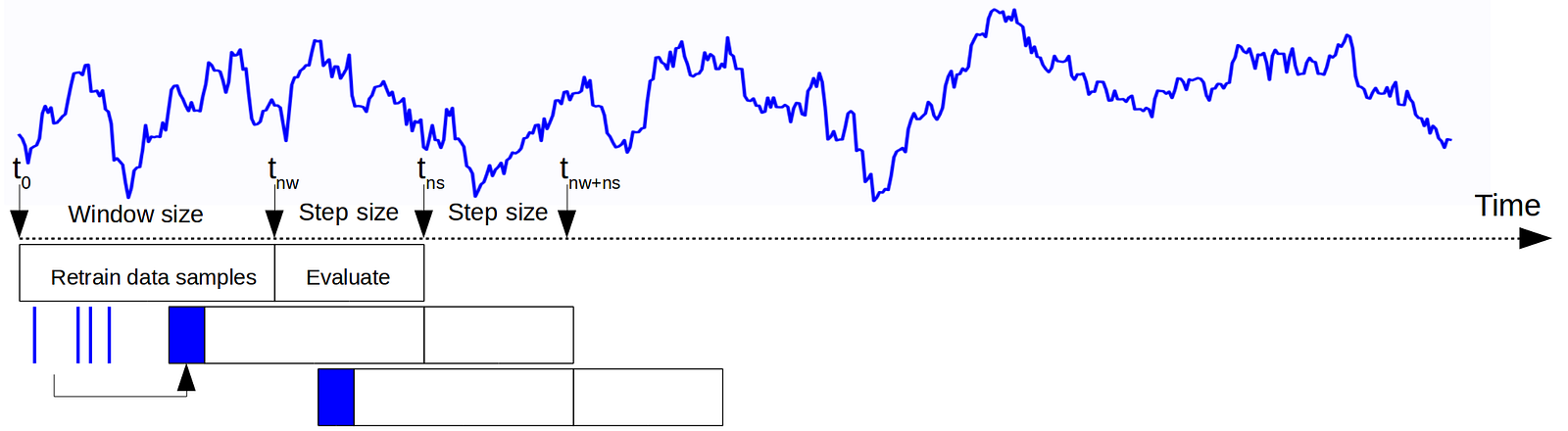Figure 2: Illustration of the continuous time-series learning setup

In our experiments, we set nw = 1000 and ns = 100, as such the model parameters are updated every 100 time steps, this is to say the prediction within the 100 time steps are generated using the fixed parameters. The underlying assumption is that for the ns consecutive data points, the same underlying distribution as the earlier nw data points is maintained. Here we need to take note that unlike other DNNs, during every batch the parameters in GPs or DGPs are retrained.

Hyper-parameter setting: Here we examine the performance of SGPR, SVGP and DDGP on financial time series prediction. The SGPR and SVGP models are implemented using the built-in functions in TensorFlow based GPflow library hyperlink. The kernel function used is the radial basis function (RBF) with 100 inducing points and 100 iterations. Here we need to emphasize that for GP models, the choice of a suitable kernel function is extremely important. The results can be very different if the kernel function is changed (for example to Matern12). The implementation of DDGP follows the work in Salimbeni, 2017. We examine two approaches, one using Adam optimizer for all DGP layers and the other with Adam optimizer for all but the final layer, which uses a natural gradient optimizer. Similar to GP model, the kernel function in DGP is also RBF, with 100 inducing points, 100 iterations and 3 layers.

Data pre-processing and handling: Before sending the raw time series into the models, we first need to reduce the non-stationary nature of the time-series, by taking the z-transform within a moving window of past 100 days price. Note the model is trying to simulate the function between x and y and function implies that with the same x, y should always be the same. Let’s say we only keep one past value in x, it is highly likely that the same x gives different y’s (i.e. using a single or current value of x may not give us an accurate estimate of its future) which violates the property of being a function. In order to deal with this we use time embedding of x, keeping more than 1 time-step information in x, e.g. past 20 time steps i.e. k = 20.

Evaluating the performance of the models

Performance measures: To evaluate the performance of different models, we look at the negative log likelihood (NLL) and symmetric mean average percentage error (SMAPE) which is defined as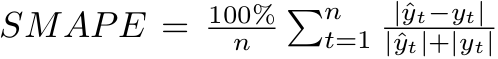These two measures evaluate the accuracy of the models. Besides the accuracy, another important measure we use is the cross correlation between the prediction and actual time series. This is to check whether a model learns a trend following behavior. If a model simply copies the exact value from the previous step, even though the reported performance in terms of NNL and SMAPE may be high, it still implies the model has not learned the underlying relation or the architecture of the model is just not suitable for prediction. To relate this measure with trend following, we compute the lag where the peak of the correlation occurs. Lag = α indicates the prediction at time t has the highest correlation with the actual time series at time t+α for all t in the testing time series on average.

Let’s now test GP and DGP models on a time series prediction task

Synthetic time series: To check whether a model works, we first test it on some synthetic data sets to rule out the influence of unexpected factors, then move on to real world ones. In terms of synthetic data sets, we will use the Mackey-Glass (MG) chaotic time series which exhibits delay induced chaotic behavior. As most time series with practical relevance are nonlinear and may exhibit chaotic signatures, MG time series serves as a good representative. The discrete time-delay MG series is generated as follows: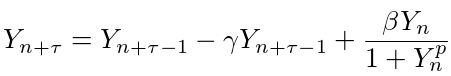τ, the delay factor is set as 30, β and γ, weights for each component are set as 0.2 and 0.1, p is the magnifying factor which is set as 10. The results for MG_{τ=30} are as follows: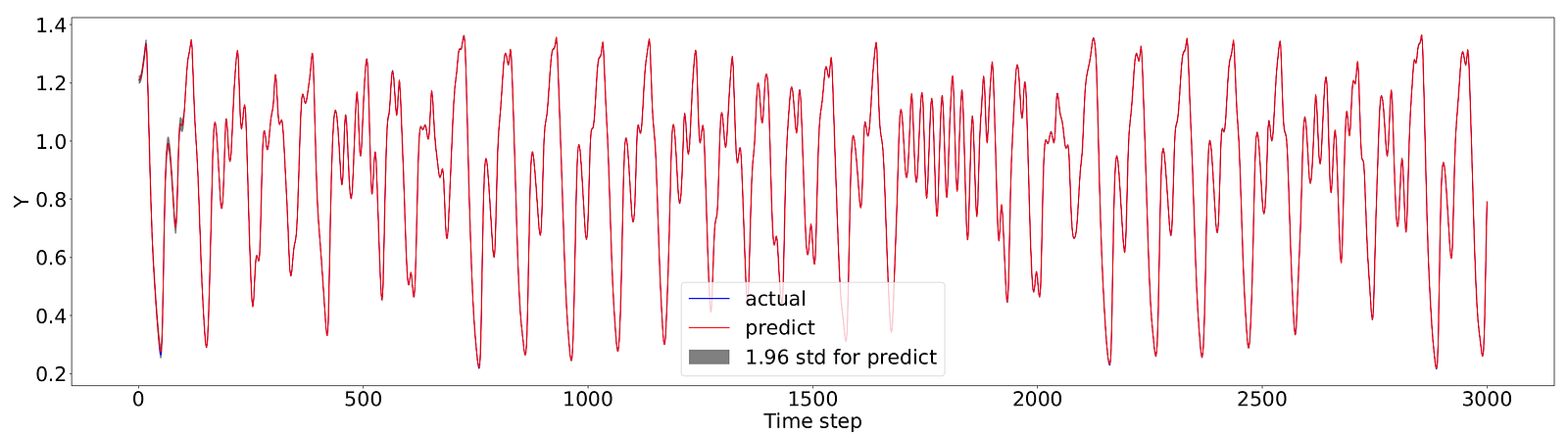Figure 3: SGPR, MG_{τ=30}, the length of the time series is 4000, overall test size is 3000. NLL: -5.36; SMAPE: 0.06%; lag: 0.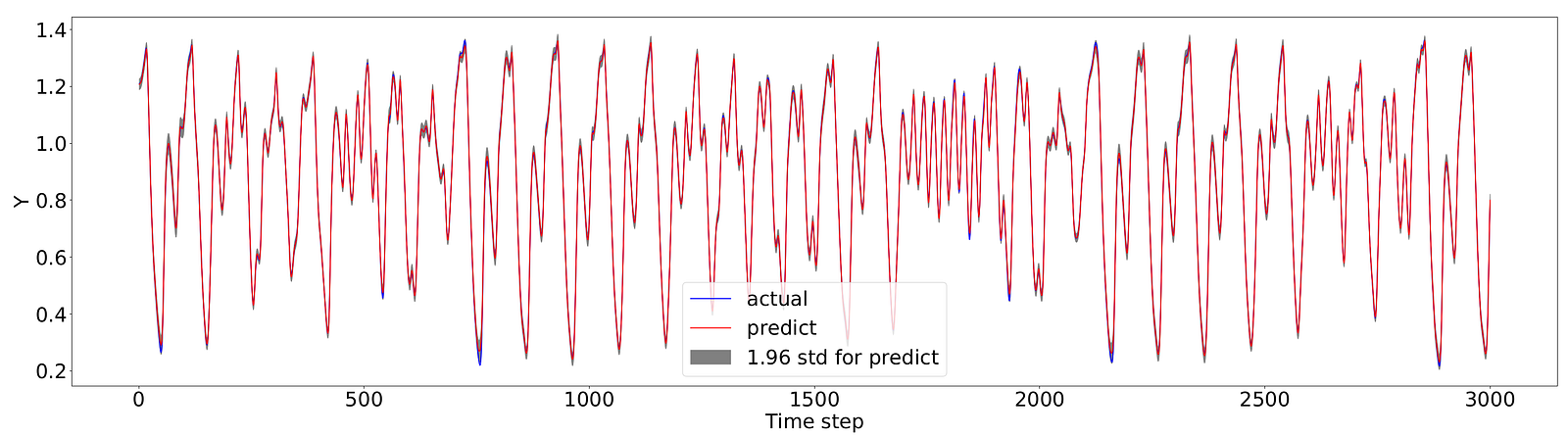Figure 4: SVGP, MG_{τ=30}, the length of the time series is 4000, overall test size is 3000. NLL: -3.39; SMAPE: 0.38%; lag: 0.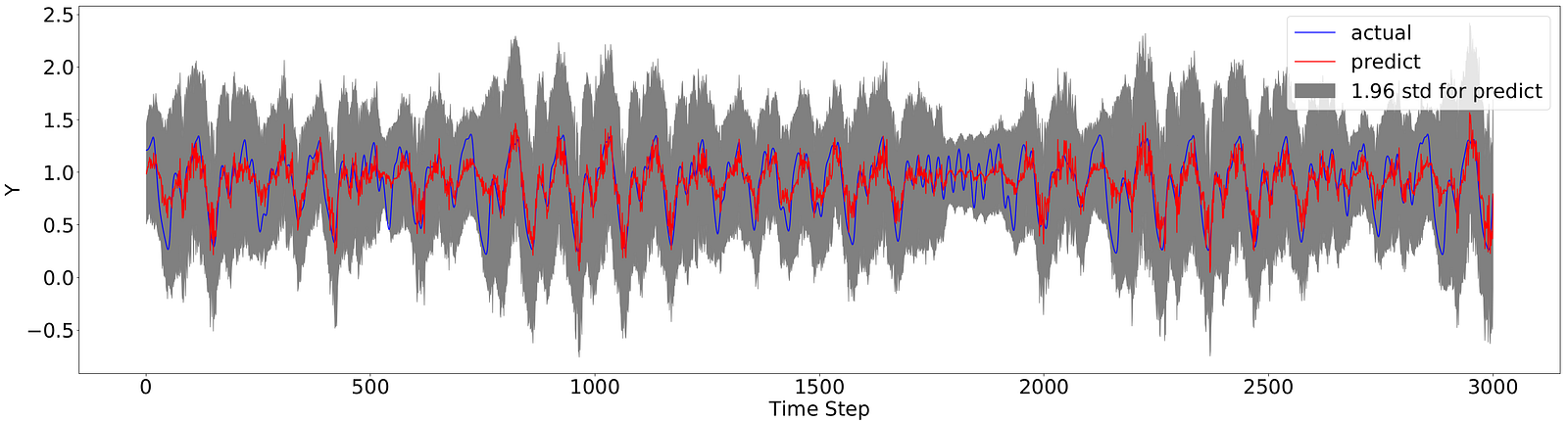Figure 5: DDGP, MG_{τ=30}, the length of the time series is 4000, overall test size is 3000. NLL: 0.003; SMAPE: 8.73%; lag: -3.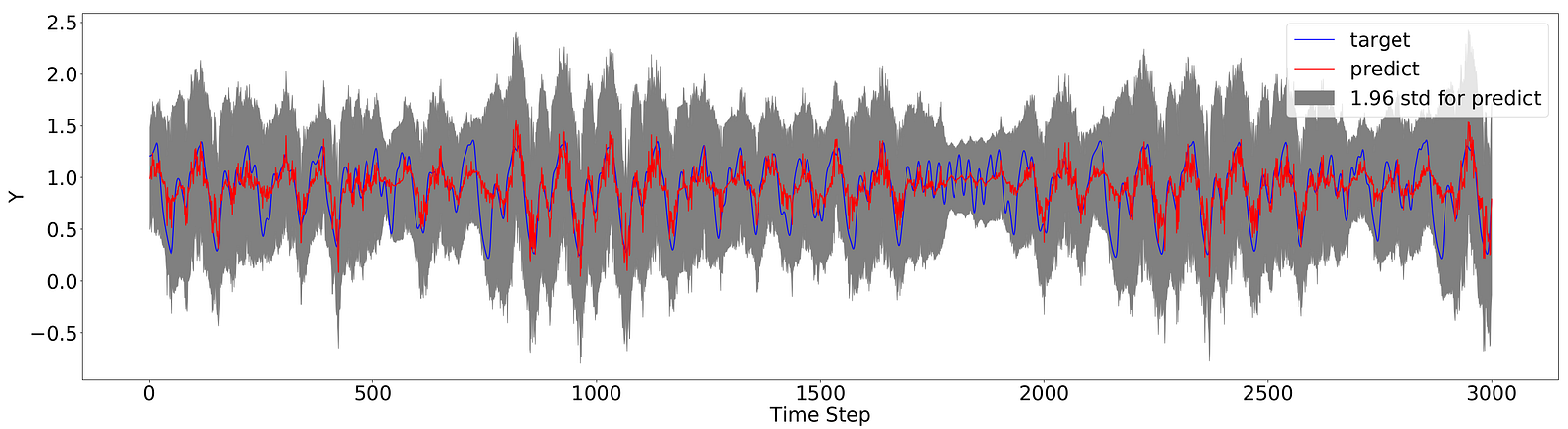Figure 6: DDGP(NG), MG_{τ=30}, the length of the time series is 4000, overall test size is 3000. NLL: 0.05; SMAPE: 4.17%; lag: -3.

Real-world time series: Now we move on to the real-world time series, we use foreign exchange (FX) with time frequency defined as a day starting from January 30 2005. Here we train the models to predict the next day price of single asset using the past 20 days price as input. The results are: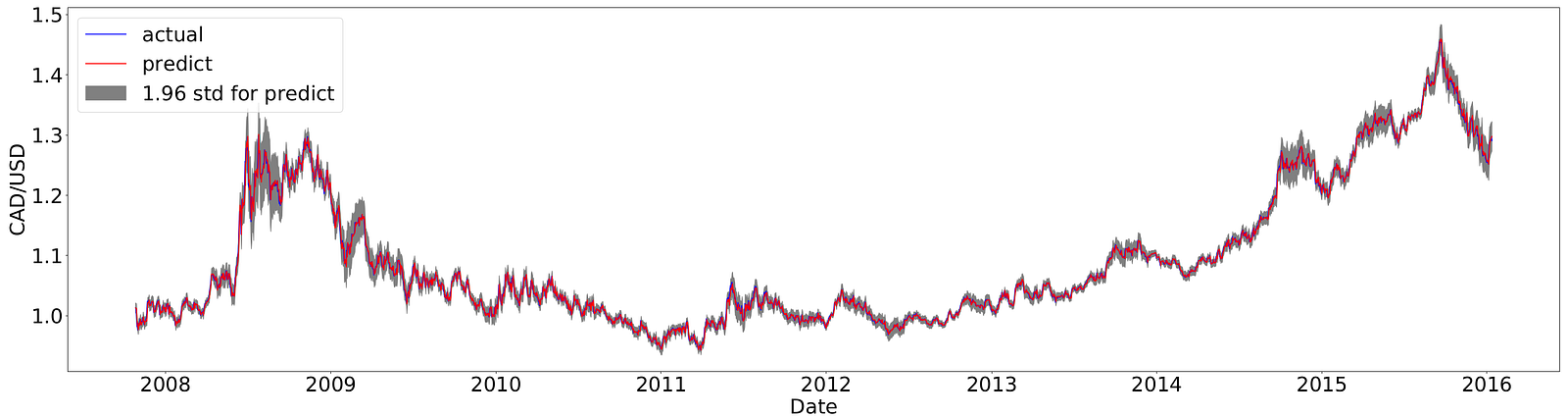Figure 7: SGPR, FX, the length of the time series is 4000, overall test size is 3000. NLL: -3.78; SMAPE: 0.18%; lag: -1.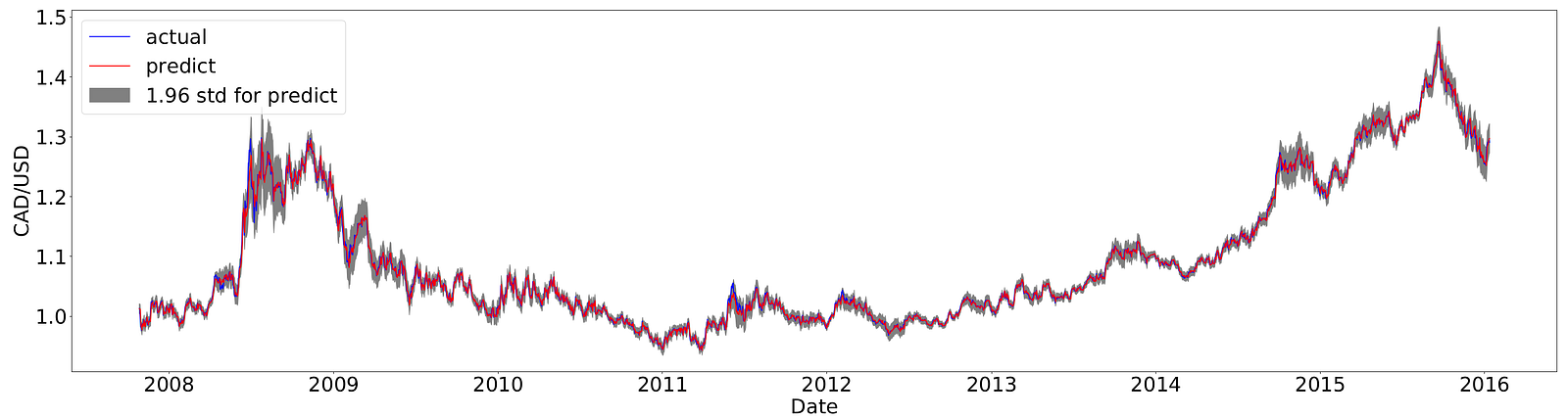Figure 8: SVGP, FX, the length of the time series is 4000, overall test size is 3000. NLL: -3.76; SMAPE: 0.19%; lag: -1.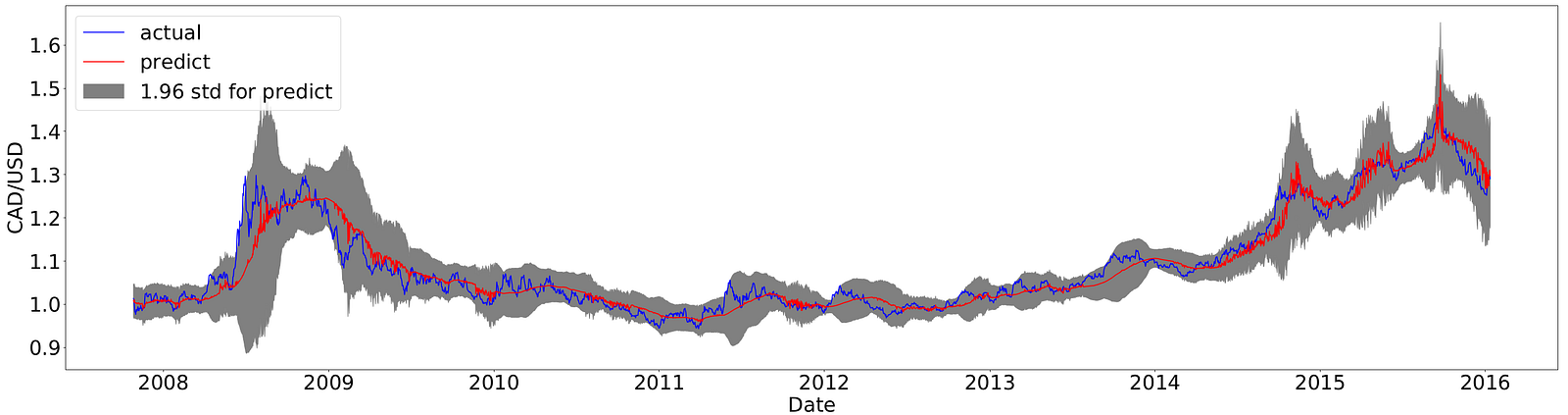Figure 9: DDGP, FX, the length of the time series is 4000, overall test size is 3000. NLL: 0.13; SMAPE: 11.90%; lag: -15.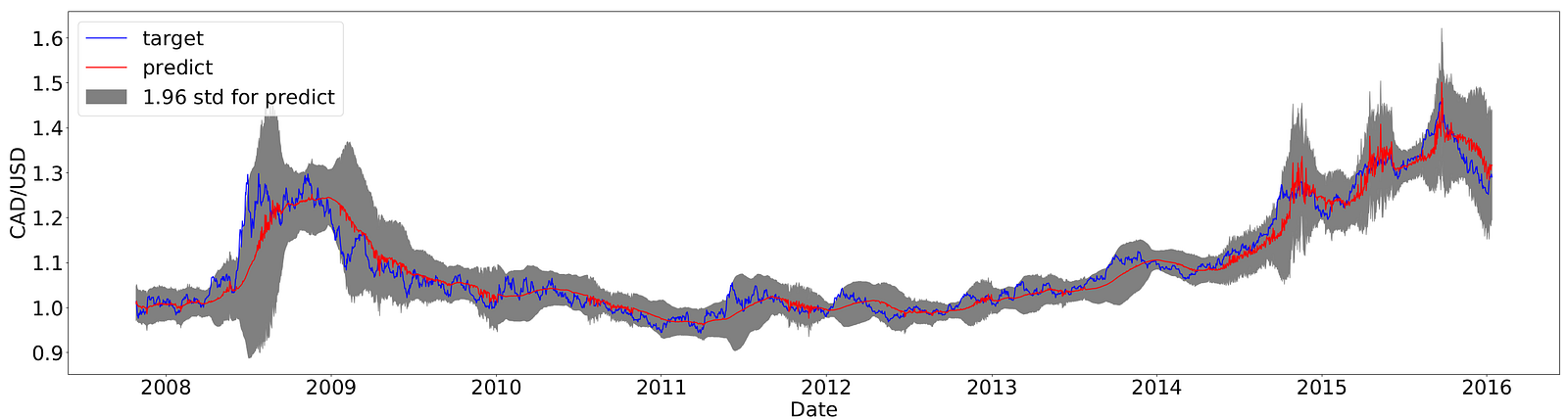Figure 10: DDGP(NG), FX, the length of the time series is 4000, overall test size is 3000. NLL: 0.18; SMAPE: 12.94%; lag: -22.

From the above figures and metrics, we could see that sparse GPs achieve the most accurate results. As we mentioned earlier, the performance of GPs relies heavily on the choice of kernel functions. In addition, the performance of SGPR and SVGP also suffers when the window size reduces.

In terms of cross correlation, for SGPR and SVGP on MG time series, the peak is at lag = 0 which shows no sign of trend following and the prediction is very accurate as it is related to the actual time series without a lag. But for FX time series, the peak occurs at lag = -1 and it indicates a one time step trend following behavior. From the zoom-in Figures 11 and 12, we can clearly see that SGPR and SVGP are just copying the value of the previous day. With this being said, this cross-correlation is not the only term to measure trend following. For DDGP and DDGP(NG), the lag peak occurs at -3 and -3 for MG_{τ=30} and -15 and -22 for FX respectively. Larger α might actually indicate the model has explored some periodic behavior in the time series, but on the other hand, α ≠ 0 also shows the prediction is bad. This cross correlation measure provides some theoretical backup for the claim of trend following, but why does this happen in single layer GPs on FX time series, but not MG time series? Our current thought is that because MG time series actually contains a very obvious pattern that the model is complex enough to learn it, but for FX time series, we may not have enough data points in the time series so that the existence of a pattern is not clear, the model then tends to just follow the trend. We will investigate more on this in a later blog post.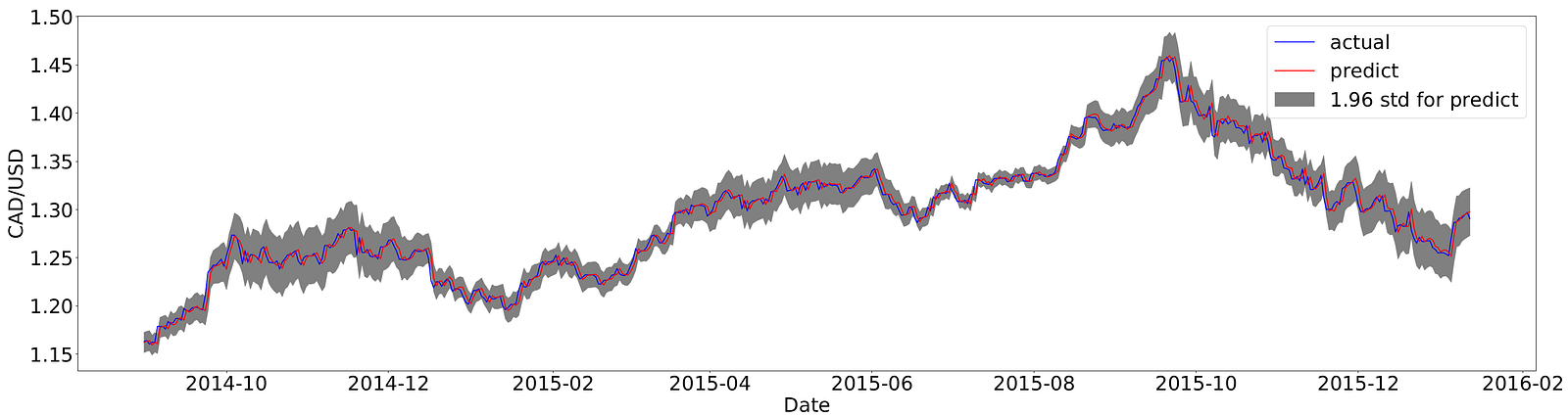Figure 11: SGPR, FX, zoom-in view of the last 500 data points, lag: 0.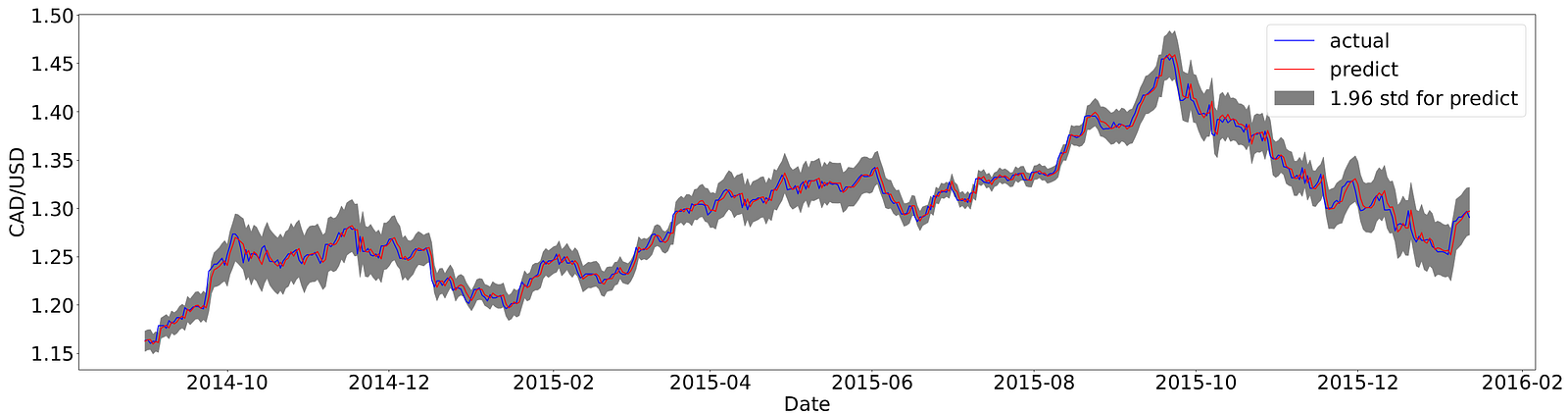Figure 12: SVGP, FX, zoom-in view of the last 500 data points, lag: 0.

This post talks about using traditional GPs and Deep GPs to predict the next step value of financial time series. Just recall the process: first we need to choose a proper prior distribution, then fit the observations in this distribution to generate a predictive distribution. This process highly depends on the choice of the prior, like we mentioned earlier, if we change the kernel function in the prior from RBF to Matern12 for the MG time series, the result is much worse. This is one of the limitations of GPs or Deep GPs. A new method which is just proposed in Aug this year, Conditional Neural Processes (CNP) and Neural Processes (NP) are actually able to get rid of the prior selection step. The idea is using a deep neural network (encoder) to generate the relationship between x and y instead of pre-defining it as of certain types. The relationship vector is then used for decoding new x into a distribution of possible y’s, here comes the bottleneck, this distribution is also assumed to be Gaussian, so the output is a mean and a standard deviation. The new algorithms CNPs and NPs can also be applied to the prediction of financial time series after some data structuring, this will appear in our later posts, if you are interested, we have a NIPS workshop paper (see reference ) discussing about the performance of GPs, deep GPs and CNPs. Cheers!

References:

 G. M. Jenkins, G. C. Reinsel and G. E. P. Box, Time Series Analysis: Forecasting and Control, Fourth Edition, John Wiley & Sons, 2008.

 C. Bishop, Pattern Recognition and Machine Learning, Spring Science+Business Media, LLC, 2006.

 A.G. de G. Matthews, M.v.d. Wilk, T. Nickson, K. Fujii, A. Boukouvalas, P. Leon-Villagra, Z. Ghahramani and J. Hensman, GPflow: a Gaussian process library using Tensorflow, in Journal of Machine Learning Research, 18, pp. 1–6, 2017.

 H. Salimbeni and M. Deisenroth, Doubly stochastic variational inference for deep gaussian processes, in Advances in Neural Information Processing Systems (NIPS), 2017.

 M. Garnelo, D. Rosenbaum, A. J. Maddison, T. Ramalho, D. Saxton, M. Shanahan, Y. W. Teh, D. J. Rezende and S. M. Eslami, Conditional neural processes, in Proceedings of the International Conference on Machine Learning (ICML), 2018.

 M. Garnelo, J. Schwarz, D. Rosenbaum, F. Viola, D.J. Rezende, S.M. Ali Eslami and Y. W. Teh, Neural processes, in Theoretical Foundations and Applications of Deep Generative Models Workshop, ICML, 2018.

 D. Teng and S. Dasgupta, Continuous time-series forecasting with deep and shallow stochastic processes, in NIPS Continual Learning 2018.

About the author: Dan is a research scientist at Neuri Pte Ltd.